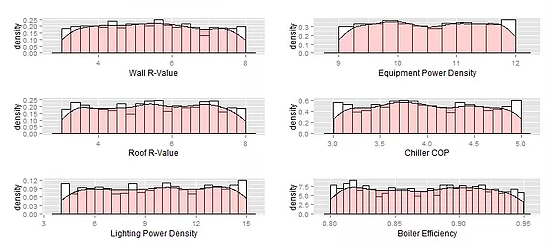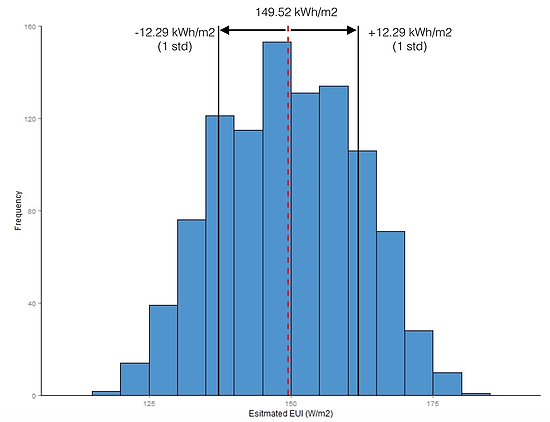top of page

# Design Parametric Study - Monte CarloWe have been hearing about Monte Carlo method a lot lately. It frequently appears in many researches. The most famous is probably the achievement from Go AI "Master" (AI "Master"), which uses the Monte Carlo tree search algorithm to evaluate every Go move.

So what is Monte Carlo method?? Here is the explanation from Wikipedia: "it is a broad class of computational algorithms that relies on repeated random sampling to obtain numerical results". but... what does this mean?

Lets demonstrate it with an example. First, we have an equation:

y = x+b

There are three variables in this equation: y, x, and b. Let's assume that we know the x is a random variable with normal distribution and b is another random variable with log-normal distribution, given both distributions have mean of 0 and standard deviation of 1.According to this information, how do we calculate y? Well, although we may be able to estimate the distribution of y using other math equations, in this case, we want to do it in a Monte Carlo way to solve the problem.

library(ggplot2) library(reshape2) x <- rnorm(1000,0,1) #randomly draw 1000 samples b <- rlnorm(1000,0,1) #randomly draw 1000 samples y <- x+b #apply the equation! mean(y) # calculate the mean of y sd(y) #calculate the standard deviation of y #start plotting! fun.test.df <- data.frame(X=x, B = b, Y = y) fun.test.df\$ID <- seq.int(nrow(fun.test.df)) fun.test.df <- melt(fun.test.df, id=c("ID")) ggplot(fun.test.df, aes(x=value, fill=variable)) + geom_density(alpha=.3)

These R codes may look very long, but the whole Monte Carlo process is only in three lines. First:

x <- rnorm(1000,0,1) #randomly draw 1000 samples b <- rlnorm(1000,0,1)#randomly draw 1000 samples

These two lines are doing the "repeated random sampling" part, and

y <- x + b # apply the equation

This line is doing the "obtain numerical results".

mean(y) # calculate the mean of y sd(y)#calculate the standard deviation of y

These two lines calculate the distribution characteristics of y, and the rest of the code is helping you generate a probability density function (PDF) plot below:Hurrah, we got our y in the image (blue area)! We can see its distribution shape, and we can also calculate its mean (1.6ish) and standard deviation (2.4ish). An equivalent python script would be:

import numpy as np x = np.random.normal(0,1,1000) #randomly draw 1000 samples for x b = np.random.lognormal(0,1,1000) #randomly draw 1000 samples for y y=x + b print "This is the mean of y: " + np.mean(y) print "This is the standard deviation of y: " + np.std(y)

The great feature about Monte Carlo is that this method solves a problem in a similar fashion as brute-force method. We do not need to understand how the equation is formed. As long as we know the inputs of the equation, and how many iterations/trials we need to conduct (we may cover this in the later post), we could successfully estimate the output.

This is particularly useful for estimating the uncertainty of any building performance metrics. For instance, most of the people treat EnergyPlus as a grey-box or black-box model, which means we do not have 100% understanding of the formulation of the energy simulation equation(s). But we may know some inputs of the model, or the design variables, such as wall insulation (r), lighting power density (lpd) and HVAC efficiency (eff) etc. If we know the uncertainty of these variables, we then can estimate the uncertainty of a building's energy consumption.

Assume you know the distribution of these variables and You want to know the possible range of the annual energy consumption. Let's formulate this equation!

EUI (kWh/m2) = f_eplus(x_r + y_lpd + z_eff...)

Based on our experience, the manual calculation is not applicable here. So the solution can only be found using Monte Carlo method. Let's do the test.

Here is the medium size office building downloaded from DOE commercial reference building. It is a three-story building, with three AHUs. Each AHU provides heating/cooling/ventilation to a floor.We defined a few design variables and describe their uncertainties:

1. Wall R-value: a uniform distribution range from R-3 to R-8 (m2K/W)

2. Roof R-value: a uniform distribution range from R-3 to R-8 (m2K/W)

3. Lighting power density (LPD): a uniform distribution range from 4 to 15 (W/m2)

4. Equipment power density (EPD): a uniform distribution range from 9 to 12 (W/m2)

5. Chiller COP: a uniform distribution range from 3 to 5

6. Boiler Efficiency: a uniform distribution range from 0.8 to 0.95

Now, let's start messing around with our medium office using Monte Carlo! We firstly random sample 1000 times from each design variable. Look at the image below; the distributions seem to cover most of the values in their specified range.

We now have 1000 samples under each design variables, and we can view their distributions!Let's export these sampled data into EnergyPlus Parametric: SetValueForRun objects and run the simulations. Since we have 1000 rows of data generated and each row contains one sample of a design variable, we need to run 1000 simulations. Once all the simulations are completed, we can extract the EUI results from the output files. Here is what we get:So, in our analysis, the building EUI is around 150 kWh/m2, with a standard deviation of 12.29 kWh/m2. The minimum EUI can reach 120 kWh/m2, and the maximum EUI is 180.4 kWh/m2... 95% confidence interval, 1st quartile...3rd quartile... you can go on and on and on because you have the whole distribution of the EUI!

Bring such information to the table will definitely provide clients and your design team extra confidence on the design.

Besides the Monte Carlo, there are many its derivatives or other methods that can help you estimate the uncertainty of building EUIs or other performance metrics. For example, the Latin Hypercube sampling, importance sampling, Markov Chain Monte Carlo, etc. We may cover some of these algorithms in the future posts.

So, now we know how to use Monte Carlo method. But why do we need Monte Carlo in building energy simulation? There are three primary reasons that I can think of:

1. Create a simulation database to learn variable correlations. Monte Carlo generates hundreds of simulations. The results collected from these simulations can be used to study how a single variable affects the building EUI, explore any correlations among the variables (e.g. WWR - window U-value, when WWR is towards 0, the window U-value / SHGC should have a negligible effect to the EUI).

2. Great way to conduct a model calibration study. Building energy model calibration is slowly adopting uncertainty in the calibration process. Techniques such as Bayesian approach calibration (here), or even simple Monte Carlo approach (here) are proved their capability in model calibration.

3. Risk assessment. Analyze the risk of the design is one of the essential parts in energy modeling. At the end of the day, we should be able to tell our clients about how much energy is used to operating the building in a year, what's the possibility of it operates lower or higher than the estimated value, and how much lower or higher it can be.

Feel free to let me know if you have anything to add on this list!

No tags yet.